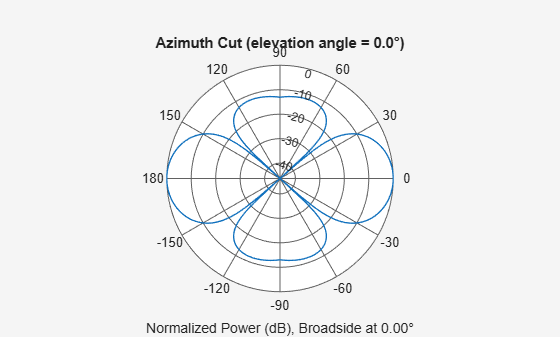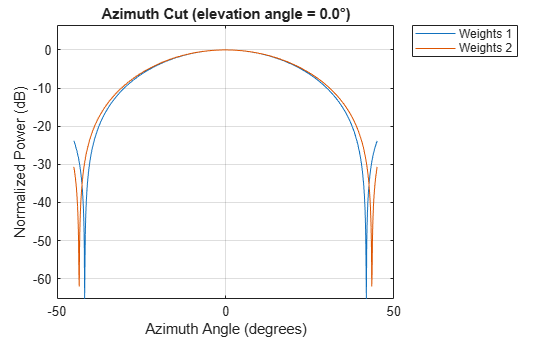# plotResponse

System object: phased.HeterogeneousURA
Package: phased

Plot response pattern of array

## Syntax

```plotResponse(H,FREQ,V) plotResponse(H,FREQ,V,Name,Value) hPlot = plotResponse(___) ```

## Description

`plotResponse(H,FREQ,V)` plots the array response pattern along the azimuth cut, where the elevation angle is 0. The operating frequency is specified in `FREQ`. The propagation speed is specified in `V`.

`plotResponse(H,FREQ,V,Name,Value)` plots the array response with additional options specified by one or more `Name,Value` pair arguments.

`hPlot = plotResponse(___)` returns handles of the lines or surface in the figure window, using any of the input arguments in the previous syntaxes.

## Input Arguments

 `H` Array object `FREQ` Operating frequency in Hertz specified as a scalar or 1-by-K row vector. Values must lie within the range specified by a property of `H`. That property is named `FrequencyRange` or `FrequencyVector`, depending on the type of element in the array. The element has no response at frequencies outside that range. If you set the `'RespCut'` property of `H` to `'3D'`, `FREQ` must be a scalar. When `FREQ` is a row vector, `plotResponse` draws multiple frequency responses on the same axes. `V` Propagation speed in meters per second.

### Name-Value Arguments

Specify optional comma-separated pairs of `Name,Value` arguments. `Name` is the argument name and `Value` is the corresponding value. `Name` must appear inside quotes. You can specify several name and value pair arguments in any order as `Name1,Value1,...,NameN,ValueN`.

`CutAngle`

Cut angle as a scalar. This argument is applicable only when `RespCut` is `'Az'` or `'El'`. If `RespCut` is `'Az'`, `CutAngle` must be between –90 and 90. If `RespCut` is `'El'`, `CutAngle` must be between –180 and 180.

Default: `0`

`Format`

Format of the plot, using one of `'Line'`, `'Polar'`, or `'UV'`. If you set `Format` to `'UV'`, `FREQ` must be a scalar.

Default: `'Line'`

`NormalizeResponse`

Set this value to `true` to normalize the response pattern. Set this value to `false` to plot the response pattern without normalizing it. This parameter is not applicable when you set the `Unit` parameter value to `'dbi'`.

Default: `true`

`OverlayFreq`

Set this value to `true` to overlay pattern cuts in a 2-D line plot. Set this value to `false` to plot pattern cuts against frequency in a 3-D waterfall plot. If this value is `false`, `FREQ` must be a vector with at least two entries.

This parameter applies only when `Format` is not `'Polar'` and RespCut is not `'3D'`.

Default: `true`

`Polarization`

Specify the polarization options for plotting the array response pattern. The allowable values are ```|'None' | 'Combined' | 'H' | 'V' |``` where

• `'None'` specifies plotting a nonpolarized response pattern

• `'Combined'` specifies plotting a combined polarization response pattern

• `'H'` specifies plotting the horizontal polarization response pattern

• `'V'` specifies plotting the vertical polarization response pattern

For arrays that do not support polarization, the only allowed value is `'None'`. This parameter is not applicable when you set the `Unit` parameter value to `'dbi'`.

Default: `'None'`

`RespCut`

Cut of the response. Valid values depend on `Format`, as follows:

• If `Format` is `'Line'` or `'Polar'`, the valid values of `RespCut` are `'Az'`, `'El'`, and `'3D'`. The default is `'Az'`.

• If `Format` is `'UV'`, the valid values of `RespCut` are `'U'` and `'3D'`. The default is `'U'`.

If you set `RespCut` to `'3D'`, `FREQ` must be a scalar.

`Unit`

The unit of the plot. Valid values are `'db'`, `'mag'`, `'pow'`, or `'dbi'`. This parameter determines the type of plot that is produced.

Unit valuePlot type
`db`power pattern in dB scale
`mag`field pattern
`pow`power pattern
`dbi`directivity

Default: `'db'`

`Weights`

Weight values applied to the array, specified as a length-N column vector or N-by-M matrix. The dimension N is the number of elements in the array. The interpretation of M depends upon whether the input argument `FREQ` is a scalar or row vector.

Weights DimensionsFREQ DimensionPurpose
N-by-1 column vectorScalar or 1-by-M row vectorApply one set of weights for the same single frequency or all M frequencies.
N-by-M matrixScalarApply all of the M different columns in `Weights` for the same single frequency.
1-by-M row vectorApply each of the M different columns in `Weights` for the corresponding frequency in `FREQ`.

`AzimuthAngles`

Azimuth angles for plotting array response, specified as a row vector. The `AzimuthAngles` parameter sets the display range and resolution of azimuth angles for visualizing the radiation pattern. This parameter is allowed only when the `RespCut` parameter is set to `'Az'` or `'3D'` and the `Format` parameter is set to `'Line'` or `'Polar'`. The values of azimuth angles should lie between –180° and 180° and must be in nondecreasing order. When you set the `RespCut` parameter to `'3D'`, you can set the `AzimuthAngles` and `ElevationAngles` parameters simultaneously.

Default: `[-180:180]`

`ElevationAngles`

Elevation angles for plotting array response, specified as a row vector. The `ElevationAngles` parameter sets the display range and resolution of elevation angles for visualizing the radiation pattern. This parameter is allowed only when the `RespCut` parameter is set to `'El'` or `'3D'` and the `Format` parameter is set to `'Line'` or `'Polar'`. The values of elevation angles should lie between –90° and 90° and must be in nondecreasing order. When yous set the `RespCut` parameter to `'3D'`, you can set the `ElevationAngles` and `AzimuthAngles` parameters simultaneously.

Default: `[-90:90]`

`UGrid`

U coordinate values for plotting array response, specified as a row vector. The `UGrid` parameter sets the display range and resolution of the U coordinates for visualizing the radiation pattern in U/V space. This parameter is allowed only when the `Format` parameter is set to `'UV'` and the `RespCut` parameter is set to `'U'` or `'3D'`. The values of `UGrid` should be between –1 and 1 and should be specified in nondecreasing order. You can set the `UGrid` and `VGrid` parameters simultaneously.

Default: `[-1:0.01:1]`

`VGrid`

V coordinate values for plotting array response, specified as a row vector. The `VGrid` parameter sets the display range and resolution of the V coordinates for visualizing the radiation pattern in U/V space. This parameter is allowed only when the `Format` parameter is set to `'UV'` and the `RespCut` parameter is set to `'3D'`. The values of `VGrid` should be between –1 and 1 and should be specified in nondecreasing order. You can set `VGrid` and `UGrid` parameters simultaneously.

Default: `[-1:0.01:1]`

## Examples

expand all

Construct a 3-by-3 heterogeneous URA with a rectangular lattice, then plot the array's azimuth response at 300 MHz.

```sElement1 = phased.ShortDipoleAntennaElement(... 'FrequencyRange',[2e8 5e8],... 'AxisDirection','Z'); sElement2 = phased.ShortDipoleAntennaElement(... 'FrequencyRange',[2e8 5e8],... 'AxisDirection','Y'); sArray = phased.HeterogeneousURA(... 'ElementSet',{sElement1,sElement2},... 'ElementIndices',[1 1 1; 2 2 2; 1 1 1]); fc = [3e8]; c = physconst('LightSpeed'); plotResponse(sArray,fc,c);```Plot the same result in polar form.

`plotResponse(sArray,fc,c,'RespCut','Az','Format','Polar');`Finally, plot the directivity.

`plotResponse(sArray,fc,c,'RespCut','Az','Unit','dbi');`Construct a square 3-by-3 heterogeneous URA composed of 9 short-dipole antenna elements with different orientations. Using the AzimuthAngles parameter, plot the array's azimuth response in the -45 degrees to 45 degrees in 0.1 degree increments. The `Weights` parameter lets you display the array's response simultaneously for different sets of weights: in this case a uniform set of weights and a tapered set.

```sElement1 = phased.ShortDipoleAntennaElement(... 'FrequencyRange',[2e8 5e8],... 'AxisDirection','Z'); sElement2 = phased.ShortDipoleAntennaElement(... 'FrequencyRange',[2e8 5e8],... 'AxisDirection','Y'); sArray = phased.HeterogeneousURA(... 'ElementSet',{sElement1,sElement2},... 'ElementIndices',[1 1 1; 2 2 2; 1 1 1]); fc = [3e8]; c = physconst('LightSpeed'); wts1 = ones(9,1)/9; wts2 = [.7,.7,.7,.7,1,.7,.7,.7,.7]'; wts2 = wts2/sum(wts2); plotResponse(sArray,fc,c,'RespCut','Az',... 'Format','Line',... 'AzimuthAngles',[-45:0.1:45],... 'Weights',[wts1,wts2],'Unit','db');```